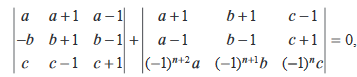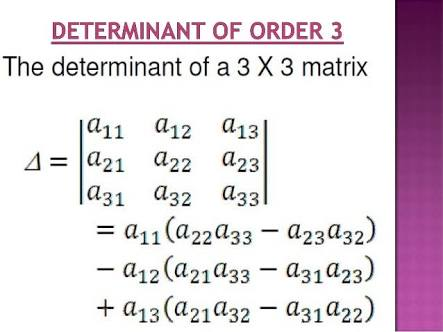## Filters

Sort by :
Clear All
Q
Engineering
120 Views   |

Let a, b, c be such that  .Ifthen the value of is

• Option 1)

any even integer

• Option 2)

any odd integer

• Option 3)

any integer

• Option 4)

zero

As we learnt in

Value of determinants of order 3 --

So n is any odd integer.

Option 1)

any even integer

Incorrect Option

Option 2)

any odd integer

Correct Option

Option 3)

any integer

Incorrect Option

Option 4)

zero

Incorrect Option

View More
Engineering
154 Views   |

Directions : Questions are Assertion- Reason type questions. Each of these questions contains two statements :

Statement- 1 (Assertion) and Statement - 2 (Reason).

Each of these questions also has four alternative choices, only one of which is the correct answer. You have to select the correct choice.

Question : Let be a  2 x 2 matrix

Statement-1 :

Statement-2 :

• Option 1)

Statement-1 is true,Statement-2 is true; Statement-2 is not a correct explanation for Statement-1

• Option 2)

Statement- 1 is true, Statement-2 is false

• Option 3)

Statement-1 is false, Statement-2 is true

• Option 4)

Statement-1 is true, Statement-2 is true; Statement-2 is correct explanation for Statement-1

As we learnt in

Property of adjoint of A -

- wherein

denotes adjoint of  and    denotes determinant  of  and  is the order of the matrix

Statement 2 is true

Statement 1 is true

Option 1)

Statement-1 is true,Statement-2 is true; Statement-2 is not a correct explanation for Statement-1

Correct Option

Option 2)

Statement- 1 is true, Statement-2 is false

Incorrect Option

Option 3)

Statement-1 is false, Statement-2 is true

Incorrect Option

Option 4)

Statement-1 is true, Statement-2 is true; Statement-2 is correct explanation for Statement-1

Incorrect Option

View More
Exams
Articles
Questions